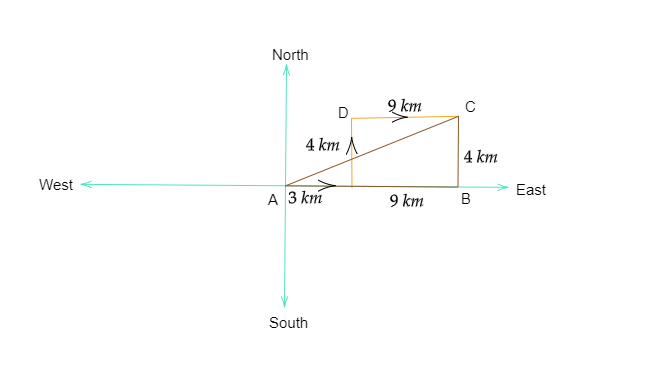# A body travels a distance of 3 km towards East, then 4 km towards North and finally 9 km towards East. What is the resultant displacement ?In the above diagram, the journey of the boy has been represented.

AC  is the displacement.

And to find the value of the displacement $(AC)$ let us use Pythagoras theorem in $\vartriangle ABC$

$AC^2=AB^2+BC^2$

Or $AC^2=(9+3)^2+4^2$

Or $AC^2=12^2+4^2$

Or $AC^2=144+16$

Or $AC^2=160$

Or $AC=\sqrt{160}$

Or $AC=12.5\ km$

Updated on: 10-Oct-2022

49 Views